# The importance of priors

## The problem

Since 2016, a lot of authors tryed to extract information on the neutrino mass ordering using cosmological data, alone and in combination with neutrino oscillation constraints.
One of the most debated topics regards the parameterization and the priors that should be used when describing the neutrino mass sector in such kind of analyses.

Simpson et al. used logarithmic priors on the three neutrino masses (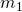,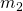,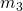) to describe the parameter space and found a strong preference for normal ordering which was never noticed in other analyses (see also Schwetz et al.). Neutrino oscillation data, however, are only sensitive to the squared-mass differences, not to the absolute values of the neutrino masses.

On the other hand, Caldwell et al. adopted a logarithmic prior on the lightest neutrino mass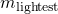and linear priors on the two squared-mass differences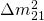and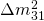, which we can naively expect to be more efficient, since it directly deals with the mass splittings that influence neutrino oscillation observables.

In our paper we explored the different possibilities and finally concluded the debate.
Naming case A the parameterization which uses the three neutrino masses (,,) and case B the one considering the lightest neutrino mass and the two squared-mass differences (,,), we studied neutrino oscillation, neutrinoless double beta decay and cosmological data using either linear or logarithmic priors. We used the Bayesian evidences of each case to determine the most efficient parameterization to deal with each dataset and derive conclusions that are not based on theoretical considerations but on data.

## Comparing parameterizations and priors

The first plot shows a comparison of the two parameterizations and using different priors, considering only neutrino oscillation data in the case of normal (left) and inverted ordering (right).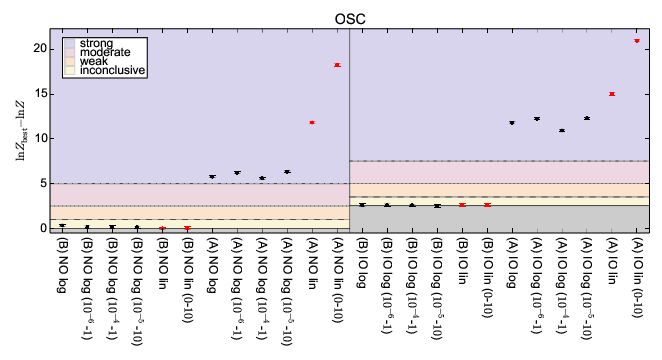As we can see, all the prior choices in case B are completely equivalent. On the other hand, case A is always strongly penalized, that is to say that case A is much less efficient to explore the parameter space of neutrino oscillations using the three mass eigenstates than using only one neutrino mass and the two mass splittings.

The result can be seen also in another way: the best prior/parameterization is the one for which the smallest fraction of initial parameter space is incompatible with data.
In case A the waste of parameter space is related to the fact that only a small fraction of parameter space is permitted: the three masses must be very close to one another according to neutrino oscillation data.
The inefficiency is also clear from the difference in computation time for the two cases, which is much higher for case A.

The second set of figures shows what happens in case B when more experimental results are included.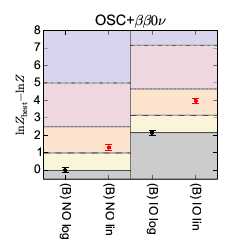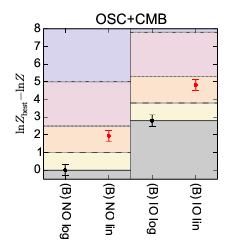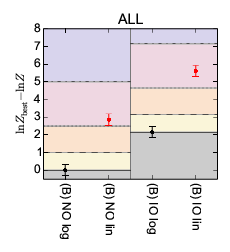Since data prefer smaller values of the lightest neutrino mass, in this case a weak or moderate preference for logarithmic prior appears. The reason is that linear priors tend to give more importance to higher values of the neutrino masses, which are excluded by data: in the linear case a larger fraction of parameter space is not permitted with respect to the logarithmic case.

As a summary, our results show that analyses including constraints from neutrino oscillations together with probes of neutrino masses are better performed using a logarithmic prior on the lightest neutrino massand linear priors on the two squared-mass differencesand.

## Neutrino mass ordering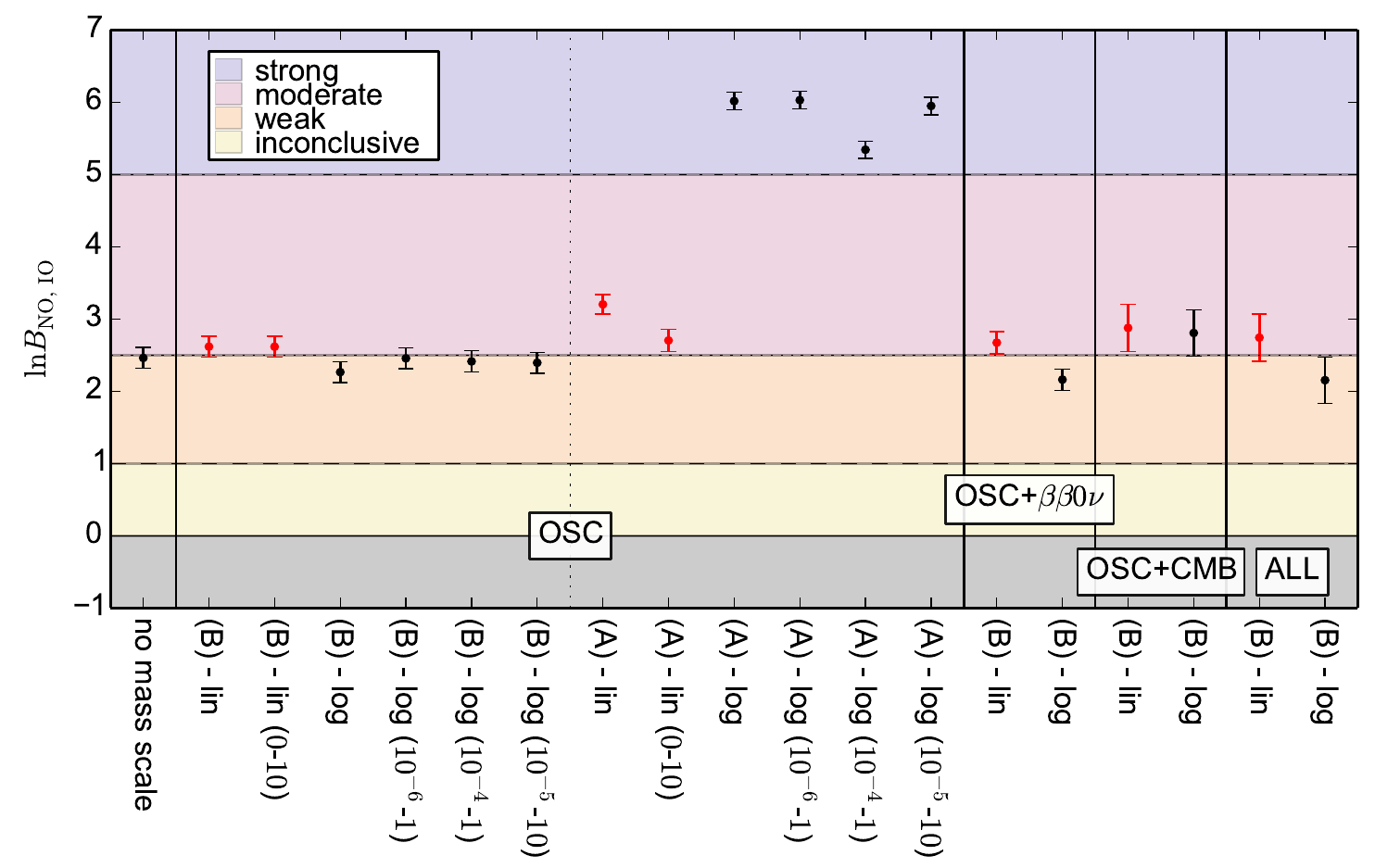Neutrino oscillation data nowadays show a preference in favor of neutrino mass ordering, as depicted by the first point in the left of this plot. The result is rather stable against changes in the parameterizations and priors, and also against the inclusion of more data from neutrinoless double beta decay or cosmology.

The only exception is represented by the four point which correspond to case A and logarithmic priors on the three neutrino masses. In this case the difference is driven by the larger allowed parameter space for the second-to-lightest mass eigenstate in normal ordering.

Please note that the results shown here are based on 2017 neutrino oscillation data. The situation has changed with more recent data, which now give a stronger preference for normal ordering, as described in this page.

Note: if you need the figures displayed in this page in PDF format, you can find them in the source archive available here.